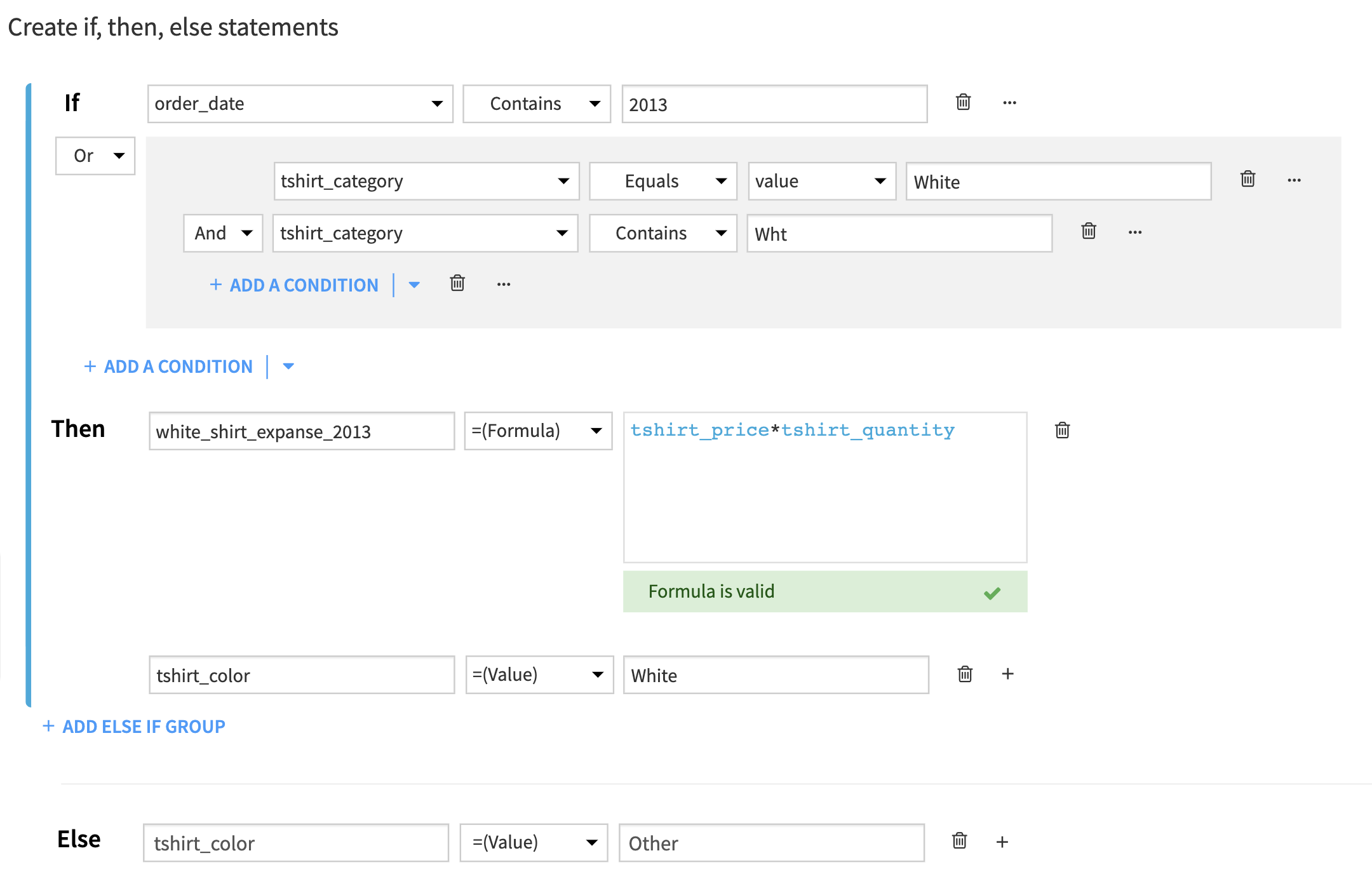# Create if, then, else statements¶

This processor performs actions or calculations based on conditional statements defined using an if, then else syntax.

IF [Condition(s)] THEN [Action(s) If True]

ELSE IF (optional) [Condition(s)] THEN [Action(s) If True]

ELSE IF (optional) [Condition(s) THEN [Action(s) If True]

ELSE (optional) [Action(s) if False]

## Conditions¶

A condition is defined with a column, an operator, a type of comparison and a value.

• Column: choose any column from the dataset.

• Operator: For example, string operators refer to operators that apply to string columns, like “contains”, “equals”, “matches the regex”; while numerical operators like “>”, “==“, apply to numerical columns. If you are not sure of what is the column type, refer to the storage type in the input dataset of the recipe, given under the name of the column.

• Type of comparison: choose to compare either to a value or to another existing column.

• Value: use an existing column or enter a value.

A Condition can be:

• Simple: Column, operator, and value or existing column.

• A conjunction: A (or|and) B - by adding a condition.

• Nested: A and (B or C) - by adding a group or turning a simple condition into a group.

## Actions¶

• Output column: use an existing column or create a new one for the output

• A type of output: value, column, formula

• The item that is assigned: a value, an existing column or a formula

For more details on how to use formulas, see the formula language documentation